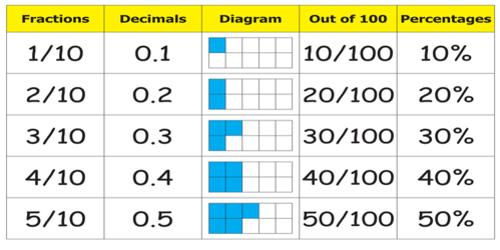Relating Fractions to Decimals

Decimals are a type of fractional number. Decimal fractions always have a denominator based on a power of 10. For examples, the decimal 0.7 is equivalent to 7/10, and the decimal 0.79 is equivalent to the decimal 79/100.

A fraction is a part of a whole. It is written with a number on top, the numerator, and a number on bottom, the denominator, with either a slanted slash or a horizontal slash between the two numbers. You can think of fractions in terms of pies. The fraction 3/4, for example, means that if you cut a piece of pie into 4 slices, then 3 of those slices are yours.

A decimal is a number with a decimal point. If you go shopping at all, you will see decimal numbers everywhere. How much does lipstick cost? \$7.99. That’s a decimal. How much do your favorite running shoes cost? \$89.99. That’s a decimal.

Decimals are a type of fractional number. The decimal 0.5 represents the fraction 5/10. The decimal 0.25 represents the fraction 25/100. Decimal fractions always have a denominator based on a power of 10.

We know that 5/10 is equivalent to 1/2 since 1/2 times 5/5 is 5/10. Therefore, the decimal 0.5 is equivalent to 1/2 or 2/4, etc.Some common Equivalent Decimals and Fractions:

• 1 and 1/10
• 2 and 1/5
• 5 and ½
• 25 and ¼
• 50 and ½
• 75 and ¾
• 0 and 1/1 or 2/2 or 1

Changing to a Fraction from a Decimal

First, let’s see how to go from a decimal to a fraction. It’s actually quite simple. Let’s try converting 7.89.

We first write the number before the decimal point down. Now we convert the number after the decimal point into a fraction. The number after the decimal point will be our top number, our numerator. Our denominator, our bottom number, will be a 1 followed by zeroes. The number of zeroes will equal the number of decimal spaces we have.

So, we count the number of digits we have after the decimal point. We count 2, so we will have 2 zeroes on the bottom following our 1. So, our decimal 7.89 turned into a fraction is 7 89/100. This is a mixed fraction.

We can turn it into an improper fraction by multiplying our whole number with the denominator and adding the numerator part of our fraction part. This will become our new numerator. So, the new numerator of 7 89/100 is 7 * 100 + 89 = 789. So, 7 89/100 turned into an improper fraction is 789/100.

See if you can turn 0.3 into a fraction. How many decimal spaces do we have? We have 1. So, how many zeroes will we have after the 1 in our denominator? We will have 1 zero. So, our fraction is 3/10.

We left our fractions as they are in both of these cases because they are already as reduced as they can be. If, however, we ended up with a fraction such as 8/10, we could reduce it to 4/5.

Information  Source: